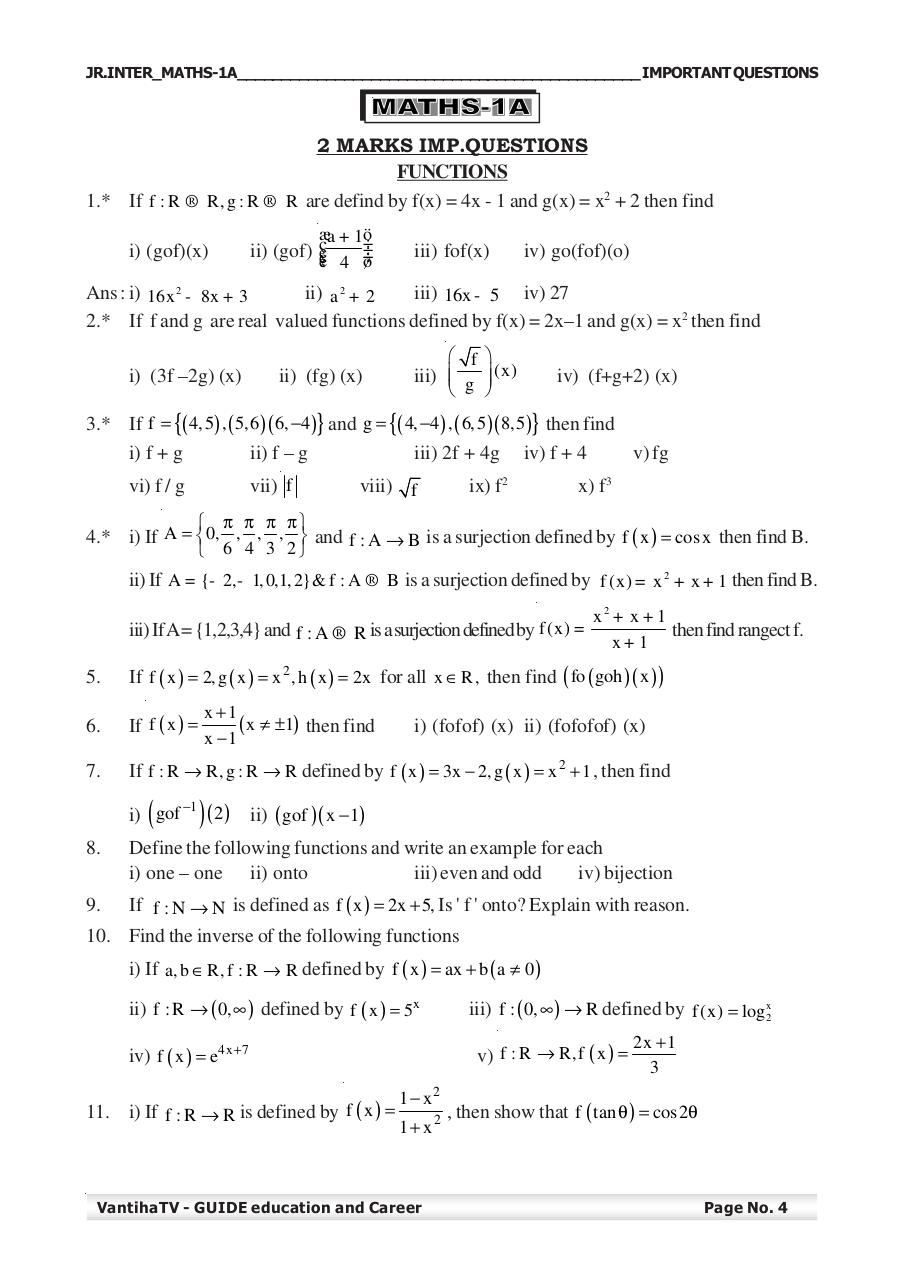# Maths 1A Chapter wise important Questions.pdfPage 1 2 3 45627

#### Text preview

JR.INTER_MATHS-1A_____________________________________________ IMPORTANT QUESTIONS

2 MARKS IMP.QUESTIONS
FUNCTIONS
1.* If f : R ® R, g : R ® R are defind by f(x) = 4x - 1 and g(x) = x2 + 2 then find
i) (gof)(x)

æa + 1ö÷
÷
ii) (gof) çççè
ø÷
4

iii) fof(x)

iv) go(fof)(o)

Ans : i) 16x 2 - 8x + 3
ii) a 2 + 2
iii) 16x - 5 iv) 27
2.* If f and g are real valued functions defined by f(x) = 2x–1 and g(x) = x2 then find
i) (3f –2g) (x)

ii) (fg) (x)

 f 

iii)  g  (x)

iv) (f+g+2) (x)

3.* If f = {( 4,5) , ( 5, 6 )( 6, −4 )} and g = {( 4, −4 ) , ( 6,5 )( 8,5 )} then find
i) f + g
ii) f – g
iii) 2f + 4g iv) f + 4
v) fg
vi) f / g

vii) f

viii) f

ix) f2

x) f3

 π π π π

4.* i) If A = 0, , , ,  and f : A → B is a surjection defined by f ( x ) = cos x then find B.
 6 4 3 2
ii) If A = {- 2, - 1, 0,1, 2}&amp; f : A ® B is a surjection defined by f (x) = x 2 + x + 1 then find B.
iii) If A = {1,2,3,4} and f : A ® R is a surjection defined by f (x) =

x2 + x + 1
then find rangect f.
x+ 1

5.

If f ( x ) = 2, g ( x ) = x 2 , h ( x ) = 2x for all x ∈ R, then find ( fo ( goh )( x ) )

6.

If f ( x ) =

7.

If f : R → R, g : R → R defined by f ( x ) = 3x − 2, g ( x ) = x 2 + 1 , then find

(

x +1
( x ≠ ±1) then find
x −1

i) (fofof) (x) ii) (fofofof) (x)

)

−1
i) gof ( 2 ) ii) ( gof )( x − 1)

8.

Define the following functions and write an example for each
i) one – one ii) onto
iii) even and odd
iv) bijection

9.

If f : N → N is defined as f ( x ) = 2x + 5, Is ' f ' onto? Explain with reason.
10. Find the inverse of the following functions
i) If a, b ∈ R, f : R → R defined by f ( x ) = ax + b ( a ≠ 0 )
ii) f : R → ( 0, ∞ ) defined by f ( x ) = 5x
iv) f ( x ) = e 4x +7

11. i) If f : R → R is defined by f ( x ) =

iii) f : ( 0, ∞ ) → R defined by f (x) = log 2x
v) f : R → R, f ( x ) =

1− x2
1 + x2

VantihaTV - GUIDE education and Career

2x + 1
3

, then show that f ( tan θ ) = cos 2θ

Page No. 4